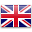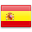PUSH Fitness & Rehabiliation
Welcome !! PUSH-as-Rx ®™ is leading the field with laser focus supporting our youth sport programs. The PUSH-as-Rx ®™ System is a sport specific athletic program designed by a strength-agility coach and physiology doctor with a combined 40 years of experience working with extreme athletes. At its core, the program is the multidisciplinary study of reactive agility, body mechanics and extreme motion dynamics. Through continuous and detailed assessments of the athletes in motion and while under direct supervised stress loads, a clear quantitative picture of body dynamics emerges. Exposure to the biomechanical vulnerabilities are presented to our team. Immediately, we adjust our methods for our athletes in order to optimize performance. This highly adaptive system with continual dynamic adjustments has helped many of our athletes come back faster, stronger, and ready post injury while safely minimizing recovery times. Results demonstrate clear improved agility, speed, decreased reaction time with greatly improved postural-torque mechanics. PUSH-as-Rx ®™ offers specialized extreme performance enhancements to our athletes no matter the age.

You’ve seen body-weight scales at stores, online, in gyms and at the doctor’s office that utilize bioimpedance analysis. These scales can be expensive and wondered what is bioimpedance analysis and is it worth the price?

Bioelectrical impedance analysis may sound complicated, however, BIA devices use today’s technology. It measures the rate at which low-level electrical current is run through the body. Based on the rate that it travels, algorithms are used to measure fat-free mass, along with other data, such as height, gender, and weight measurements to determine your body fat percentage.

• There are different types of devices, but each device requires two points of contact.
• Handheld devices use two points, which are the hands (called hand-hand BIA).
• Typical BIA scale uses the feet (called foot-foot BIA).
• You place each foot on a pad and the current travels through your body between the feet.
• There are also hand-to-foot BIA devices.
• There are many brands that make different types of BIA scales (also called bioimpedance scales)
• Newer models link with a smartphone app so you can track your progress.
• Prices of a BIA scale depend on the sophistication of the equipment.
• Some scales use multiple frequencies and more advanced algorithms, as well.
• Some provide segmental fat analysis, which means, you can get body fat measurements for each arm, leg, and belly.
• There are reports that segmental fat analysis (utilizing hand-foot BIA) are more accurate because hand-hand devices focus on measuring the upper body.
• Foot-foot scales primarily measure the lower body.
• These devices are considered safe for most people. Except:
• Bioelectrical impedance analysis should not be used by anyone with an electronic medical implant, (e.g. heart pacemaker).
• ot be used by pregnant women.
• Studies have shown that bioelectrical impedance analysis is an accurate method for measuring body fat.
• But these studies generally do not test the scales from the store.
• Experts agree the accuracy of the measurement depends on the quality of the equipment.

### (R) Resistance

• Resistance and reactance are terms from physics, which are part of the field of materials and the effects on electricity. In reality, resistance and reactance are easy to understand.
• Resistance is the ratio of electrical potential (voltage) to the current in a material. Put simply, a material with high resistance needs a high potential to generate a given amount of current in the material. A material with low resistance requires a low potential to produce the same amount of current in the material.
• The easiest way to remember is:
• Material with low resistance conducts well.
• Material with high resistance conducts poorly.
• When material conducts, it releases energy in the form of heat.
• The resistance of a material is related to the material’s ability to dissipate energy.
• Units of resistance are called ohms.
• In the human body
• Low resistance is associated with large amounts of fat-free mass.
• High resistance is associated with smaller amounts of fat-free mass.

#### The Case

• Primary conductor in the human body is ionized water.
• As the percentage of body weight that is water increases, the conductivity of the body increases.
• Body water is contained solely in fat-free mass
• Conductivity of body is proportional to amount of fat-free mass.

#### Resistance is Measured by

• A small current is applied through the body.
• The potential required to generate the current is measured.
• The ratio of potential and current along with a process called correlation and integration are used to determine the resistance and reactance.
NOTE: This alternating current resistance is not the same resistance that can be measured by a standard store ohmmeter.

### (X) Reactance

• Reactance: the effect on an electrical current caused by a material’s ability to store energy.
• A time delay between applied electrical potential and current.
• Material that stores energy easily causes high reactance, and causes a large delay in the current.
• Material that stores energy poorly has low reactance and causes a small delay in the current.
• Example: Water poured onto top of a sponge will flow out of the bottom after a delay.
• Large sponge will cause a large delay in the flow of water out the bottom
• Small sponge would cause a small delay.
• Current flows in material the same way.
• The delay flow of current from storage is the reactance.
• Units of reactance are ohms.
• In the human body:
• High reactance: large amounts of body cell mass (intracellular mass).
• Low reactance: small amounts of body cell mass.

#### The Case

• Cell membranes consist of a layer of nonconductive lipophilic material interposed between two layers of conductive molecules.
• Behave like tiny capacitors and store energy.
• Reactance in the body reflects the strength of capacitance.
• Intact cellular membranes contained primarily within body cell mass
• Reactance of the body is proportional to the amount of body cell mass

#### Reactance is Measured by

• Small current is applied through the body.
• The potential required to generate the current is measured.
• Ratio of potential and current along with process correlation and integration used to determine the reactance.

#### (Z) Impedance

• Total impedance (Z): The vector sum of effects of resistance and reactance on a current in the human body.
• Technically, impedance is the ratio of potential (V) to current (I) and is used in bioimpedance analysis.

#### How R, X, & Z Relate

Mathematical relationship between Resistance (R), Reactance (X), and Impedance (Z) are:
Z = sqrt (X2+R2)
X = Z * sin (α)
R = Z * cos (α)
phase angle = arcsin (X/Z)
phase angle = arctan (X/R)

#### (α) Phase Angle

• Phase angle is indicator of cellular health and integrity.
• Research has shown the relationship between phase angle and cellular health is increasing and nearly linear.
• Low phase angle: Consistent with inability of cells to store energy and
• Indication of a breakdown in cellular membranes.
• High phase angle: Consistent with large quantities of intact cell membranes and body cell mass.
• Phase angle reflects the ratio of body cell mass to fat-free mass.
• Phase angle is proportional to the ratio of reactance and resistance (proportional body cell mass to fat-free mass.)

#### Phase Angle Increase

• Increase in body cell mass relative to fat-free mass.
• Increase in fat-free mass relative to body weight.
• Improved hydration of fat-free mass

#### Phase Angle: Useful For Comparing

• Reactance along with the patient’s weight indicates an absolute amount of body cell mass (BCM).
• Reactance is best applied when comparing test results in a single patient at different times.
• Two patients with exact reactance (X) can have different amounts of BCM, depends on patient’s weight.
• Any patient with a higher phase angle will always have a higher proportion of BCM than patient with low phase angle.
• Phase angle indicates number of intact cell membranes.
• Phase angle does not include statistical regression analysis effect.
• Phase angle is direct measurement of relative amounts of intact cellular membranes.

#### Phase Angle Works How?

• A bioimpedance analyzer applies 50-kilohertz alternating current to the body.
• Phase angle is delay in time between the electric potential and current.
• Oscilloscope connected to the body appears as delay between the voltage waveform and current waveform.
• The period of each wave at 50 kilohertz is 20 microseconds. If, for example, the time delay is ten percent of the
• period, then the time delay is 2 microseconds.
• When expressed in units of time, it is said that the phase delay is 2 microseconds.
• Time delay can be seen as percentage of entire wave period in degrees.
• Complete wave period consists of 360 degrees. If time delay is one-tenth the total period of the wave, it is equivalent to 36 degrees.
• When time delay is expressed this way (in degrees of total wave period),
• This is the phase angle.
• When electrical potential and current are depicted sweeping around a circle
• Instead of moving over time
• The relationship between reactance, resistance, and phase angle is easier to see.
• Shown below:
• Range of phase angle in the human body is 1 to 20 degrees.
• Phase angle is the arctangent of (X/R)

#### References:

• Kyle UG, et al. Fat-Free and Fat Mass Percentiles in 5225 Healthy Subjects Aged 15 to 98 Years. Nutrition, 17:534-541, 2000.
• Mattar J, et al. Application of total body bioimpedance to the critically ill patient. New Horizons 1995, Volume 4, No, 4: 493-503.
• Ott M, et al. Bioelectrical impedance analysis as a predictor of survival in patients with human immunodeficiency virus infection. Journal of Acquired Immune Deficiency Syndrome and Human Retrovirology 1995: 9:20-25.ENES